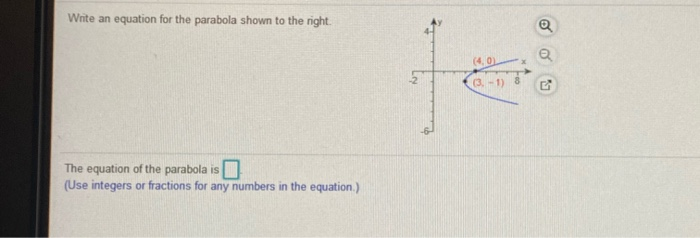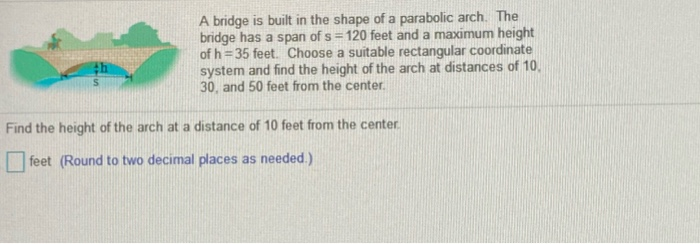# Please solve both thanks Write an equation for the parabola shown to the right. a (4.0...

###### Question:

Please solve both thanksWrite an equation for the parabola shown to the right. a (4.0 ( 3-1) The equation of the parabola is (Use integers or fractions for any numbers in the equation)
A bridge is built in the shape of a parabolic arch. The bridge has a span of s = 120 feet and a maximum height of h = 35 feet. Choose a suitable rectangular coordinate system and find the height of the arch at distances of 10 30, and 50 feet from the center, Find the height of the arch at a distance of 10 feet from the center feet (Round to two decimal places as needed.)